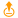# Rollup

 Block Group: Table Operations Icon:The Rollup block returns a new table that contains one row for each date and time interval in the input table. The output table contains one column for the date and time interval and one column for the value.

For information on using dataflow blocks, see Dataflow.

For answers to some common questions about working with tables, see Tables.

## Input/Output Properties

The following properties of the Rollup block can take input and give output.

• input (table)
• interval (string)
• dateColumn (string)
• valueColumn (string)
• valueRollup (enum)
• roundTime (boolean)

input receives the table in which you want to roll up values.

interval specifies the duration of the range to roll up. interval can be one of the following:

• One minute
• Five minutes
• Ten minutes
• Fifteen minutes
• Twenty minutes
• Thirty minutes
• Hour
• Two hours
• Three hours
• Four hours
• Six hours
• Twelve hours
• Day
• Two days
• Week
• Month
• Year

dateColumn specifies the name of the table column that holds the dates.

valueColumn specifies the name of the table column that holds the values to be rolled up.

valueRollup specifies the type of rollup to use. The value of the valueRollup property can be one of the following:

• First: Returns the first value for the interval.
• Last: Returns the last value for the interval.
• Average: Returns the average of all number values for the interval. Non-number values are treated as null and are not included in the calculation.
• Sum: Returns the sum of all number values for the interval. If there are no number values, returns 0.
• Max: Returns the greatest number value from the interval. If there are no number values, returns null.
• Min: Returns the smallest number value for the interval. Non-number values are treated as null and are not included in the calculation.
• Count: Returns the number of values that exist for the interval. Null values are not included.
• Concat: Returns all values for the interval, separated by commas. Duplicate values are included.
• ConcatUnique: Returns all unique values for the interval, separated by commas. Duplicate values are not included.

roundTime specifies whether to force all intervals to start at a round number.

• TRUE: Intervals start at a round number. For example, if interval is set to day, all intervals start at midnight. If interval is set to five minutes, all intervals start at multiples of five minutes past the hour.
• FALSE: The first interval starts at the first timestamp, and intervals are appended accordingly. For example, if interval is set to day and the first value is 2015/07/19T08:01:19, then all intervals begin at T08:01:19.

columnFilterType - specifies which columns will be processed.

• Whitelist: only specified columns are processed.
• Blacklist: only columns not specified above are processed.

Resulting tables contains all the columns from the input table. It doesn't cut off columns that are not processed.

## Output Property

The following property of the Rollup block can give output but cannot take input.

• output (table)

output returns the rolled up table.

## Example

The following image shows an example of the Rollup block. In this example, the output table contains the sum value for each week.